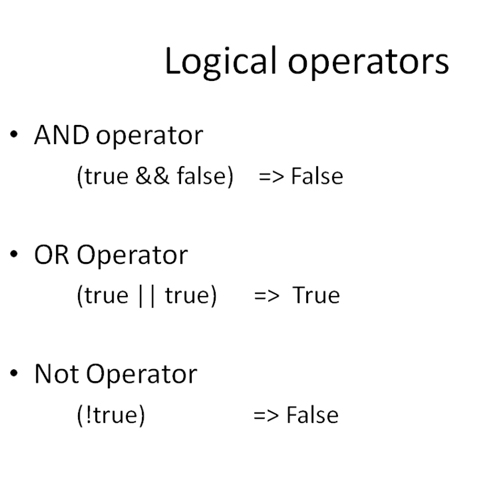## What are Basic Operators in Java

Arithmetic operators in java must be familiar to you because they function exactly as they do in basic mathematics. The one that is new is % which divides one operand by another and returns the remainder as its result.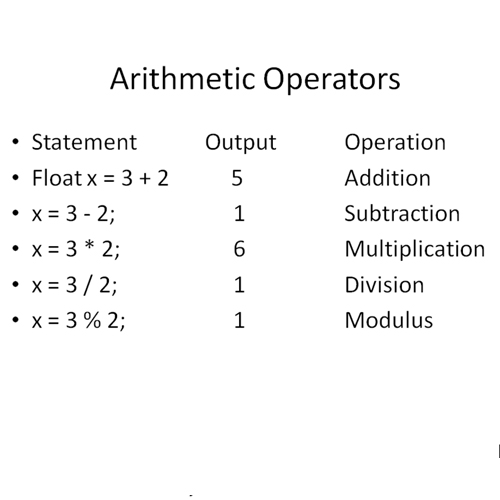Assignment Operators:

Simple assignment operator shifts the value of the operands on right side of “=” to the variable on the left side.

They can be used in combination with the arithmetic operators. They include following:

+= Add AND assignment operator, It adds right operand to the left operand and assign the result to left operand
-= Subtract AND assignment operator, It subtracts right operand from the left operand and assign the result to left operand
*= Multiply AND assignment operator, It multiplies right operand with the left operand and assign the result to left operand
/= Divide AND assignment operator, It divides left operand with the right operand and assign the result to left operand
%= Modulus AND assignment operator, It takes modulus using two operands and assign the result to left operand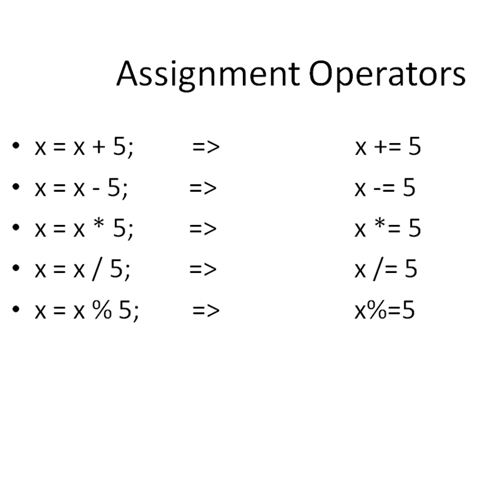Assignment Operators as Unary Operators:

++ Incrementing Operator: ++ operator increments the value of a variable by one.
Ex: if the value of x is 5 then after doing x++ its value will become 6.
Pre increment: Using ++ operator before variable (++x) means its value is updated first and then updated value is used.
Ex: if value of x ==5 so doing y = ++x; the value of y becomes 6.
Post increment: Using ++ operator after variable (x++) means its value is used first then incremented.
Ex: if value of x is 5 so doing y = x++; the value of y becomes 5, but x is incremented to 6.

— Decrementing Operator: — operator decrements the value of variable by one.
Ex: if the value of x is 5 then after doing x– its value will become 4.
Pre Decrement: Using — operator before variable (–x) means its value is decremented first and then decremented value is used.
Ex: if value of x ==5 so doing y = –x; the value of y becomes 4 and x is decremented to 4.
Post decrement: Using “- -“ operator after variable (x–) means its value is used first then decremented.
Ex: if value of x is 5 so after y = x–; the value of y remains 5, but x is decremented to 4.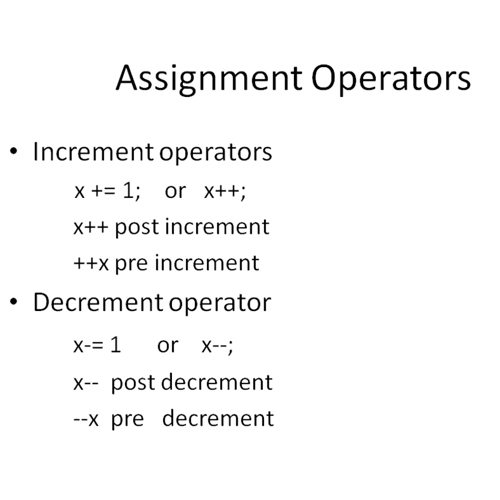Comparison Operators:

These operators in java are used to compare two values or variables and return the true or false condition. Following type of comparison operators are used in java:
== Equal to : Returns true if two values are equal. Else return false
!= Not equal to: Returns true if two values are not equal. Else returns false.
greater then Greater than: Returns true if the value on the left side is greater than value on the right
greater or equal Greater than or equal to : Returns true of the value on the left side is greater or equal to right.
less than Less than : Returns true if the value on the left side is less than value on the right.
less than or equal Less than or equal to : Returns true of the value on the left side is less than or equal to right.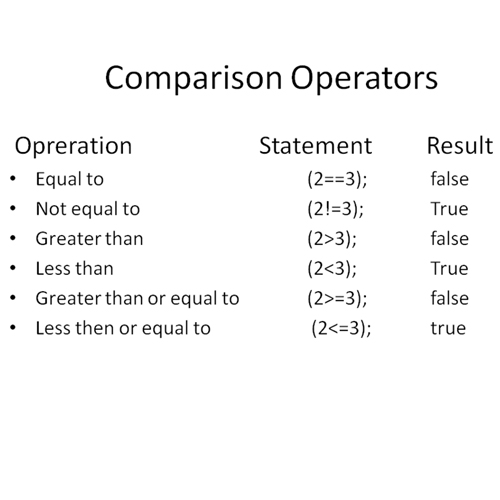Logical Operators:

&& And operator: Returns true if conditions on both sides are true. If one condition is false then it returns false.
|| OR operator: Returns true if either of the one condition is true. If both are true it returns true.
! NOT Operator: Returns the reversed condition.
Ex: (!true) returns false.